# AQA A Level Physics复习笔记2.1.1 Atomic Structure

### Atomic Structure

• The atoms of all elements are made up of three types of particles: protons, neutrons and electrons.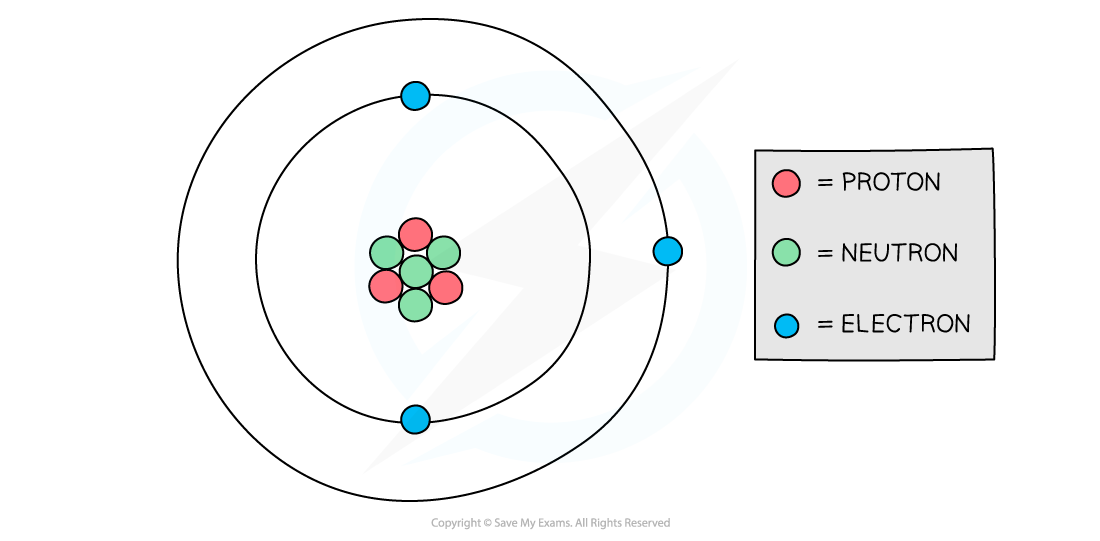Protons and neutrons are found in the nucleus of an atom while electrons orbit the nucleus

• The properties of each particle in SI units are shown in the table below: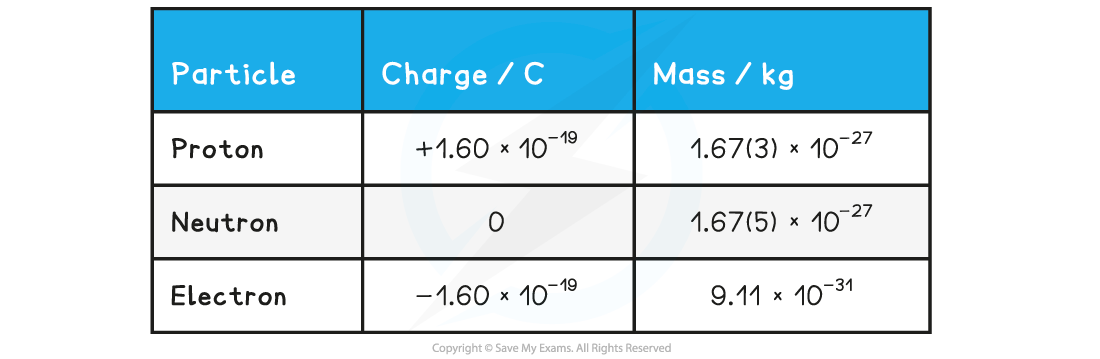• The relative properties of each particle are shown in the table below: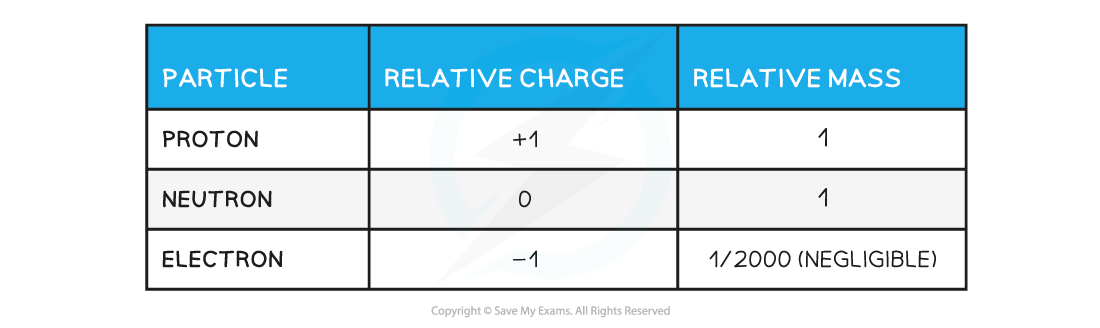• A stable atom is neutral (it has no charge)
• Since protons and electrons have the same charge, but opposite signs, a stable atom has an equal number of both for the overall charge to remain neutral

#### Exam Tip

Remember not to mix up the ‘atom’ and the ‘nucleus’. The ‘atom’ consists of the nucleus and electrons. The ‘nucleus’ just consists of the protons and neutrons in the middle of the atom, not the electrons.

### Specific Charge

• In physics ‘specific’ means a characteristic of a property of a specific substance ie. per unit mass, length, area, volume etc.
• For example, specific heat capacity refers to the thermal energy per unit mass of a substance

• In the same way, the specific charge of a particle is the ratio of its charge to its mass, which can be calculated using the equation:• Specific charge is measured in units of coulombs per kilogram (C kg–1)
• Values for the specific charge of the electron and proton are given on the datasheet as the ‘charge / mass ratio’
• The specific charge of the electron = (e / me) = 1.76 × 1011 C kg–1
• The specific charge of the proton = (e / mp) = 9.58 × 107 C kg–1

#### Calculating Specific Charge

• You may be asked to find the specific charge of an ion or a nucleus
• Charge of a proton or electron, e = 1.60 × 10–19C
• Mass of a proton, mp (or neutron, mn) = 1.67 × 10–27 kg

• To calculate the specific charge of an ion:
• Charge = Total number of electrons added / removed × (1.60 × 10–19 C)
• Mass = Total number of nucleons × (1.67 × 10–27 kg)

• To calculate the specific charge of a nucleus:
• Charge = Total charge of the protons × (1.60 × 10–19 C)
• Mass = Total number of nucleons × (1.67 × 10–27 kg)

• The number of nucleons is given by the mass number of the ion or nucleus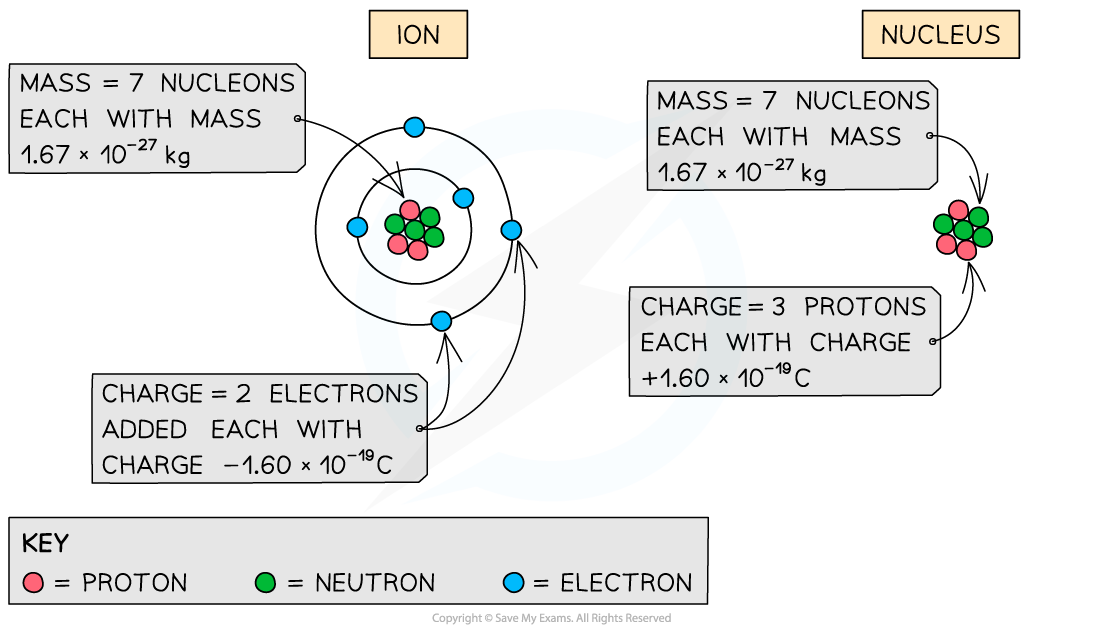How the total charge and mass are calculated depends on whether you are calculating the specific charge for an ion or a nucleus

#### Worked Example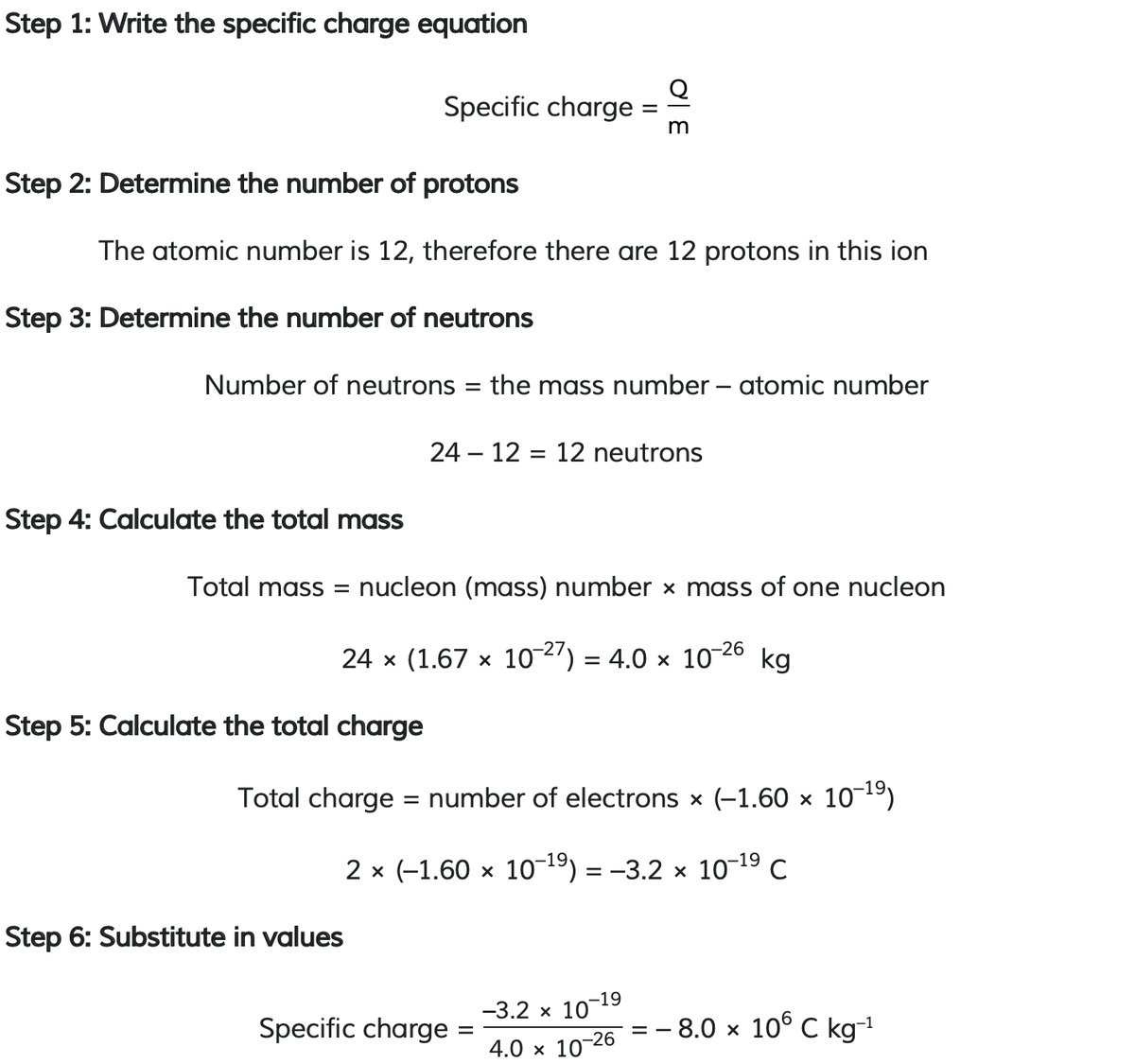#### Exam Tip

Sometimes you might be asked if the specific charge is positive or negative for an ion. Since the electron has a negative charge, the rules are as follows:

• If there is a gain in electrons, the specific charge will be negative.
• If there is a loss of electrons, the specific charge will be positive.